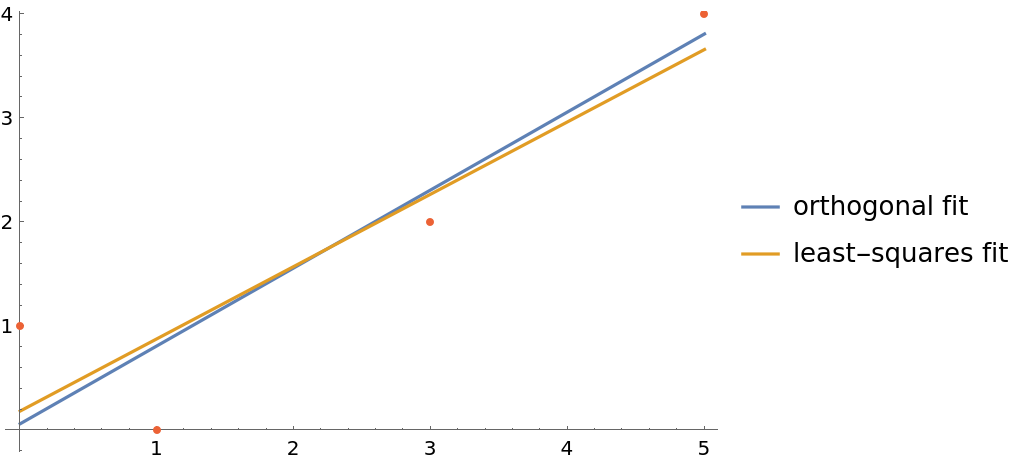#Function Repository Resource:

# OrthogonalLineFit

Find the best fitting line with respect to orthogonal distance

Contributed by: Jan Mangaldan
 ResourceFunction["OrthogonalLineFit"][data] finds the best fitting orthogonal distance regression line to data.

## Details

Orthogonal distance regression is also known as orthogonal least squares or total least squares.
The orthogonal distance regression line is the line for which its orthogonal distance from the given data is minimized.
ResourceFunction["OrthogonalLineFit"] returns an InfiniteLine object.

## Examples

### Basic Examples (2)

Parameters for a 2D line:

 In:=Out=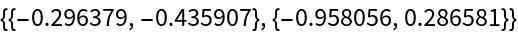Generate some points near the line:

 In:=Out=Find the orthogonal distance regression line:

 In:=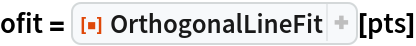Out=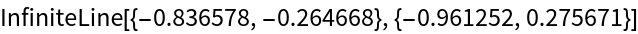Compare the regression line and the true line with the data:

 In:=Out=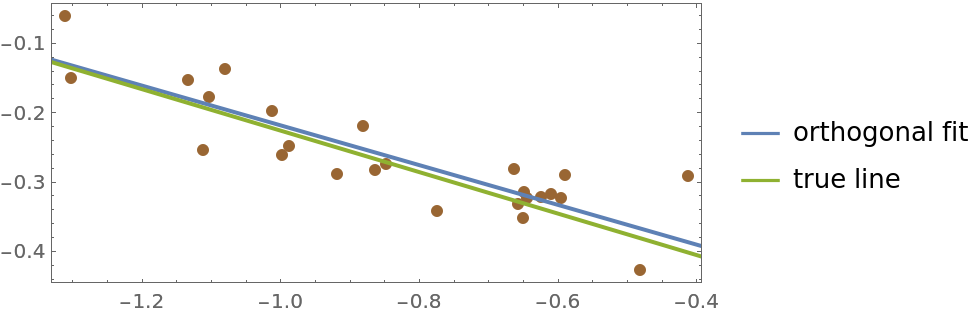Parameters for a 3D line:

 In:=Out=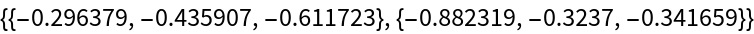Generate some points near the line:

 In:=Out=Find the orthogonal distance regression line:

 In:=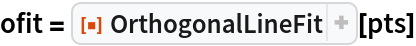Out=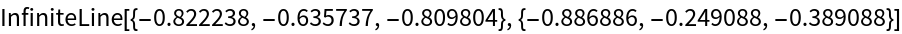Compare the regression line and the true line with the data:

 In:=Out=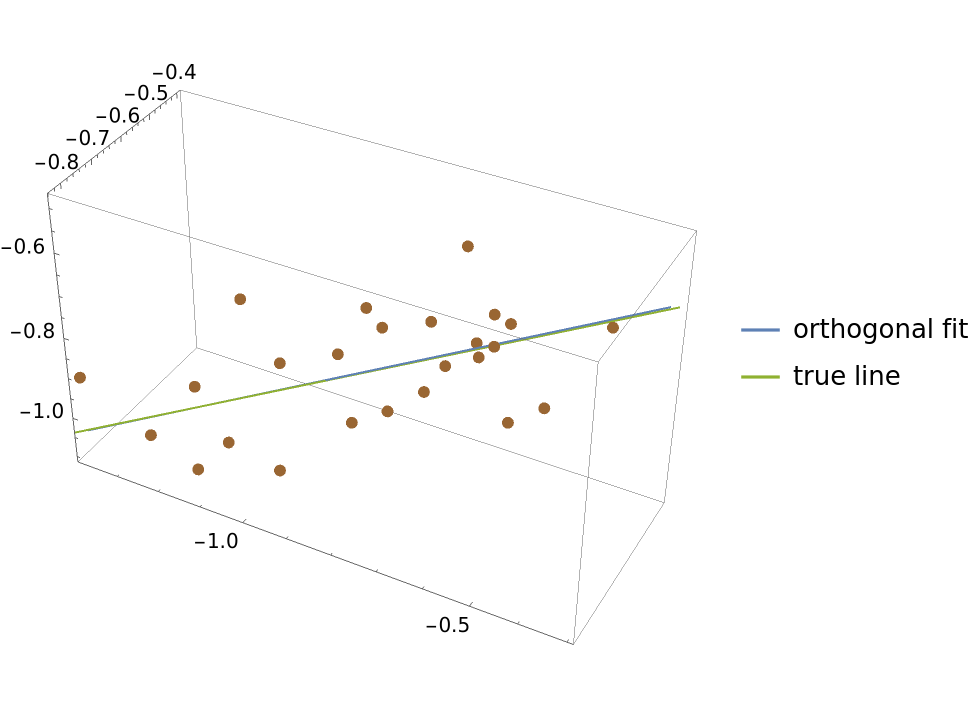### Scope (2)

Here is a list of values:

 In:=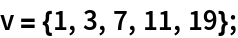When coordinates are not given, OrthogonalLineFit assumes the values are to be paired up with 1,2,:

 In:=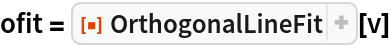Out=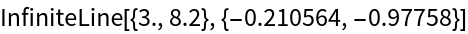In:=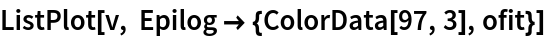Out=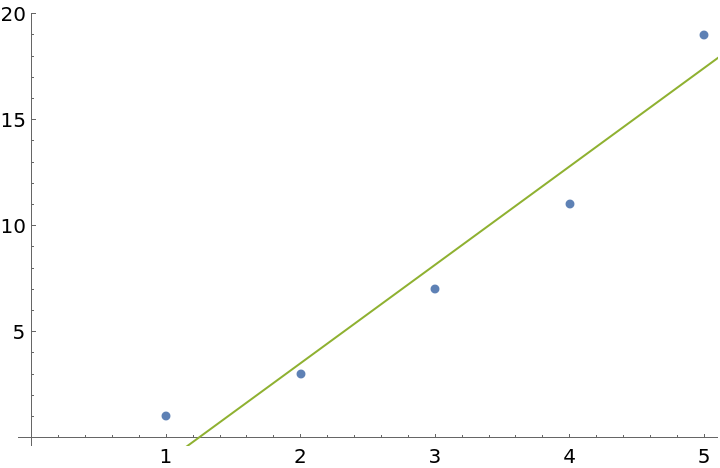### Properties and Relations (6)

Here is some data:

 In:=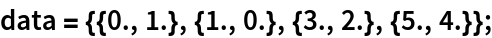This computes the parameters for the orthogonal distance regression line using the definition:

 In:=Out=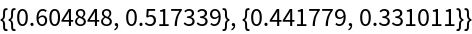In:=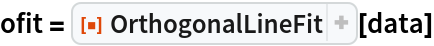Out=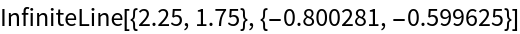Use RegionMember to compare the equations for the best fit line generated by both methods:

 In:=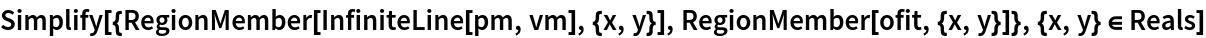Out=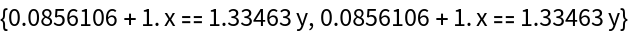Construct a function from the orthogonal fit:

 In:=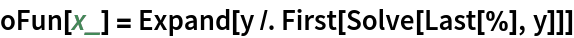Out=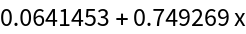Compare the orthogonal fit with the conventional least-squares fit:

 In:=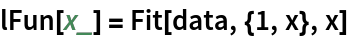Out=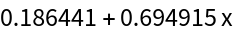In:=Out=